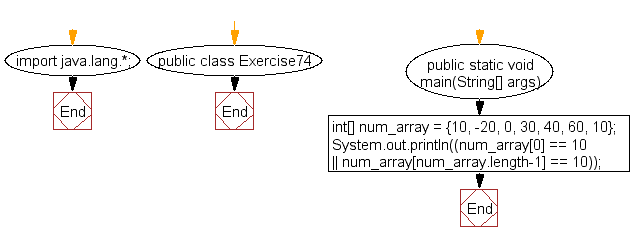﻿ Java exercises: Test if 10 appears as either the first or last element of an array of integers - w3resource# Java Exercises: Test if 10 appears as either the first or last element of an array of integers

## Java Basic: Exercise-74 with Solution

Write a Java program to test if 10 appears as either the first or last element of an array of integers. The length of the array must be greater than or equal to 2.

Sample Solution:

Java Code:

``````import java.lang.*;
public class Exercise74 {
public static void main(String[] args)
{
int[] num_array = {10, -20, 0, 30, 40, 60, 10};
System.out.println((num_array == 10 || num_array[num_array.length-1] == 10));
}
}
```
```

Sample Output:

```true
```

Pictorial Presentation:Flowchart:Java Code Editor:

What is the difficulty level of this exercise?

﻿

## Java: Tips of the Day

initial:

Returns all the elements of an array except the last one. Use Arrays.copyOfRange() to return all except the last one

```public static <T> T[] initial(T[] elements) {
return Arrays.copyOfRange(elements, 0, elements.length - 1);
}
```

Ref: https://bit.ly/3zEKw9u